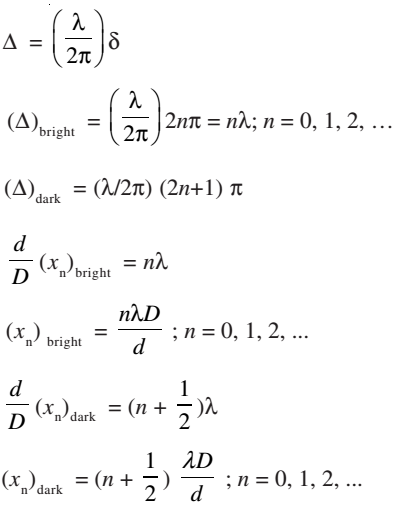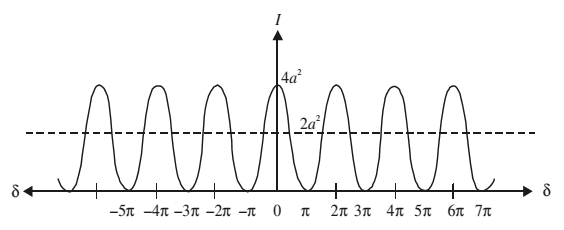# Young’s Double Slit Experiment

In this experiment, sunlight was allowed to pass through a pin hole Sand then, at some distance away, through two pin holes S1 and S2 equidistant from Sand close to each other.

According to Huygens’ wave theory of light, spherical wavefronts would spread out from the pin hole S which get divided into two wavefronts by S1 and S2. If S is illuminated by a monocromatic source of light (such as sodium), these act as coherent sources and in-phase waves of equal amplitude from these sources superpose as they move beyond S1S2. As a consequence of superposition (of the two sets of identical waves from S1 and S2), redistribution of energy takes place and a pattern consisting of alternate bright and dark fringes is produced on the screen such as placed at C.### Constructive Interference

From the superposition principle, some points on the screen C have maximum displacement (or amplitude) because the crests due to one set of waves coincide with the crests due to another set of waves. At this point, the waves arrive in-phase and hence the total amplitude is much higher than the amplitude of individual waves. The same holds true for the points where the troughs due to one set of waves coincide with the troughs due to another set. Such points will appear bright because the intensity of light wave is proportional to the square of the amplitude. Superposition of waves at these points leads to constructive interference.

### Destructive Interference

The points where the crests due to one set of waves coincide with the troughs due to the other set and vice-versa, the total amplitude is zero. It is so because the waves reach these points completely out of phase. Such points appear dark on the screen. These points correspond to destructive interference.

### Intensity of Fringes

The phenomenon of interference arises due to superposition of two harmonic waves of same frequency and amplitude but differing in phase. Let the phase difference between these two waves be δ.

y1 = a sin ωt

y2 = a sin (ωt + δ)

According to the principle of superposition of waves, the resultant displacement is given by

y = y1 + y2

= a sin ωt + a sin (ωt + δ)

= a[sin ωt + sin (ωt + δ)]

= 2a sin (ωt + δ/2) cos (–δ/2)

= A sin (ωt + δ/2)

where amplitude of the resultant wave is given by

A = 2a cos (δ/2)

The intensity of the resultant wave at point P is expressed as

I ∝ A2

I ∝ 4a2 cos2 (δ/2)

To see the dependence of intensity on the phase difference between the two waves, consider the following two cases:

Case 1: When the phase difference, δ= 0, 2π, 4π, …, 2nπ

I = 4a2 cos2 0

I = 4a2

Case 2: When, δ = π, 3π, 5π, ……, (2n+1)π

I = 4a2 cos2 (δ/2)

I = 0

When phase difference between superposing waves is an integral multiple of 2π, the two waves arrive at the screen in-phase and the resultant intensity (or brightness) at those points is more than that due to individual waves (which is equal to 4a2). On the other hand, when phase difference between the two superposing waves is an odd multiple of π, the two superposing waves arrive at the screen out of phase. Such points have zero intensity and appear to be dark on the screen.

### Phase Difference and Path Difference

To know whether a point on the screen will be bright or dark, you need to know the phase difference between the waves arriving at that point. The phase difference can be expressed in terms of the path difference between the waves during their journey from the sources to a point on the observation screen. Waves starting from S1 and S2 are in phase. Thus, whatever phase difference arises between them at the point P is because of the different paths traveled by them up to observation point from S1 and S2.

∆ = S2P – S1P

Path difference of one wavelength is equivalent to a phase difference of 2π. Thus, the relation between the phase difference δ and the path difference ∆ is

∆ = (λ/2π)δ

Bright fringes (corresponding to constructive interference) are observed when the phase difference is 2nπ and dark fringes are observed when the phase difference is (2n+1)π.

∆ = S2P – S1P = S2A = d sin θ

Assuming θ to be small,

sin θ ≈ tan θ ≈ θ

sin θ = x/D

∆ = d sin θ = d(x/D)### Fringe Width

Fringe width, β is given by

β = (x3)bright – (x2)bright = λD/d

The fringe width of an interference pattern remains the same for any two consecutive value of n. The fringe width is directly proportional to linear power of wavelength and distance between the source plane and screen and inversely proportional to the distance between the slits.